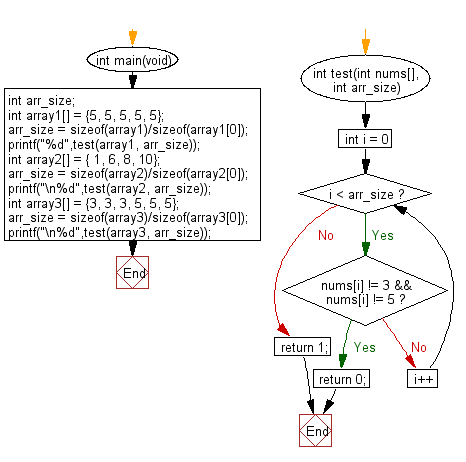﻿ C Program: Check if an array contains a 3 or a 5# C Exercises: Check if a given array of integers contains a 3 or a 5

## C-programming basic algorithm: Exercise-58 with Solution

Write a C program to check if a given array of integers contains a 3 or a 5.

C Code:

``````#include <stdio.h>
#include <stdlib.h>
int main(void){
int arr_size;
int array1[] = {5, 5, 5, 5, 5};
arr_size = sizeof(array1)/sizeof(array1);
printf("%d",test(array1, arr_size));
int array2[] = { 1, 6, 8, 10};
arr_size = sizeof(array2)/sizeof(array2);
printf("\n%d",test(array2, arr_size));
int array3[] = {3, 3, 3, 5, 5, 5};
arr_size = sizeof(array3)/sizeof(array3);
printf("\n%d",test(array3, arr_size));
}

int test(int nums[], int arr_size)
{
for (int i = 0; i < arr_size; i++)
{
if (nums[i] != 3 && nums[i] != 5) return 0;
}
return 1;
}
``````

Sample Output:

```1
0
1
```

Pictorial Presentation:Flowchart:C Programming Code Editor:

What is the difficulty level of this exercise?

Test your Programming skills with w3resource's quiz.

﻿

## C Programming: Tips of the Day

Why doesn't a+++++b work?

printf("%d",a+++++b); is interpreted as (a++)++ + b according to the Maximal Munch Rule!.

++ (postfix) doesn't evaluate to an lvalue but it requires its operand to be an lvalue.

! 6.4/4 says the next preprocessing token is the longest sequence of characters that could constitute a preprocessing token"

Ref : https://bit.ly/3fdldUT